# Trigonometric Formulae

Go back to  'Trigonometry'

## Introduction

Let’s consider a right-angled triangle. There are $$3$$ sides, which are the Hypotenuse, the Opposite side and the Adjacent side. The longest side is known as the Hypotenuse, the side opposite to the angle is the Opposite side and the side where both hypotenuse and opposite side rests is the Adjacent side.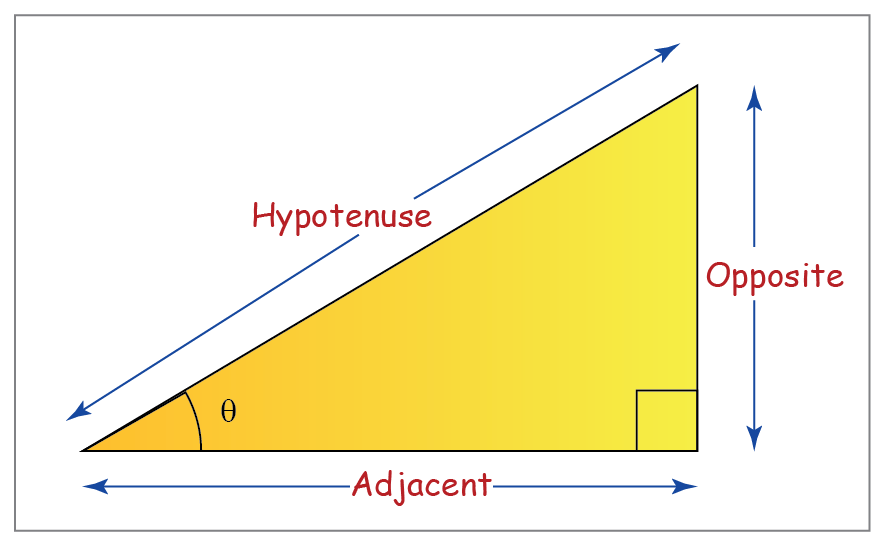## Trigonometric Functions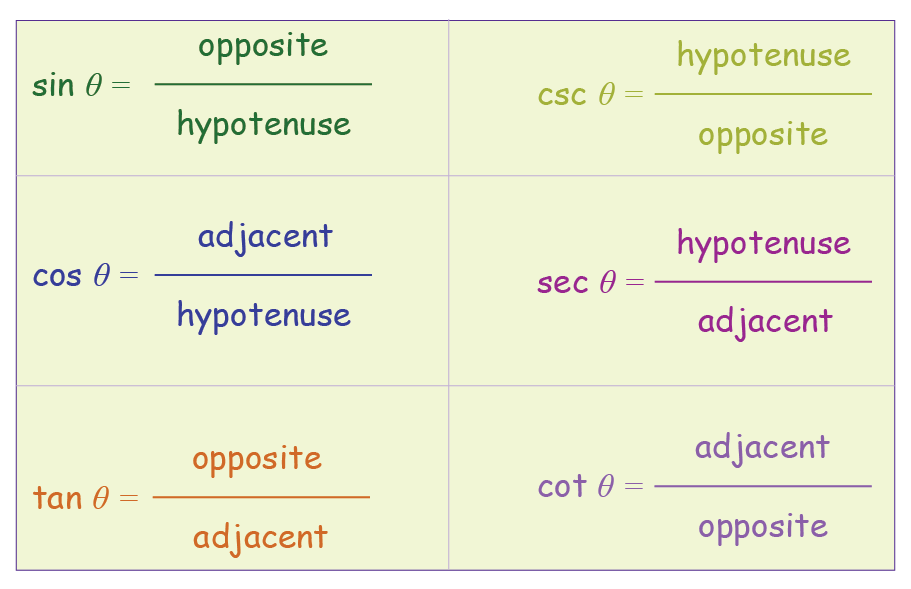## Complementary angles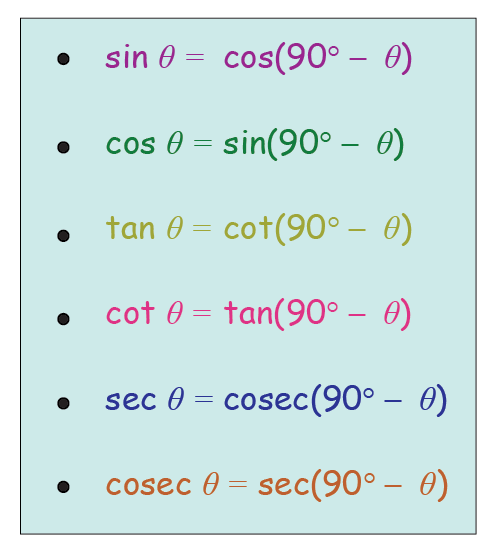## Reciprocal Identities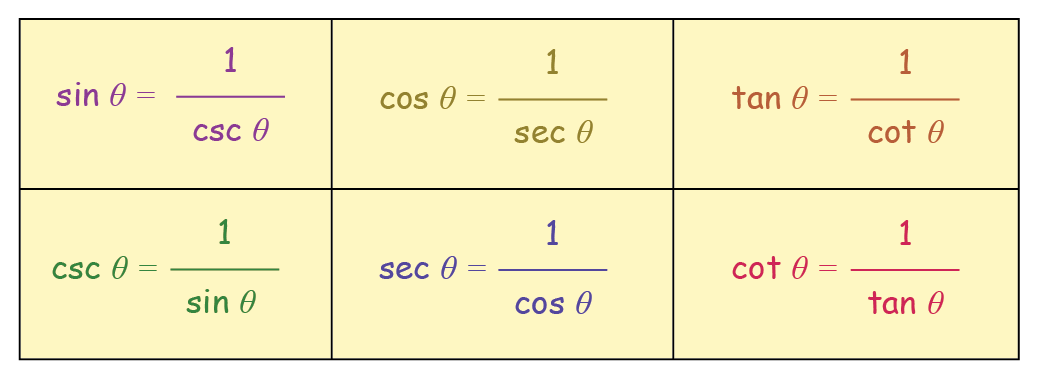## Pythagorean Identities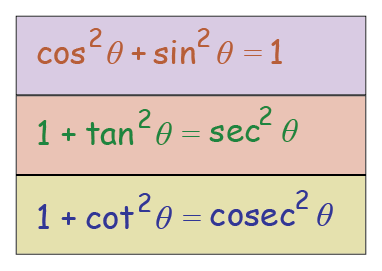## Inverse trigonometric functions with their Range  values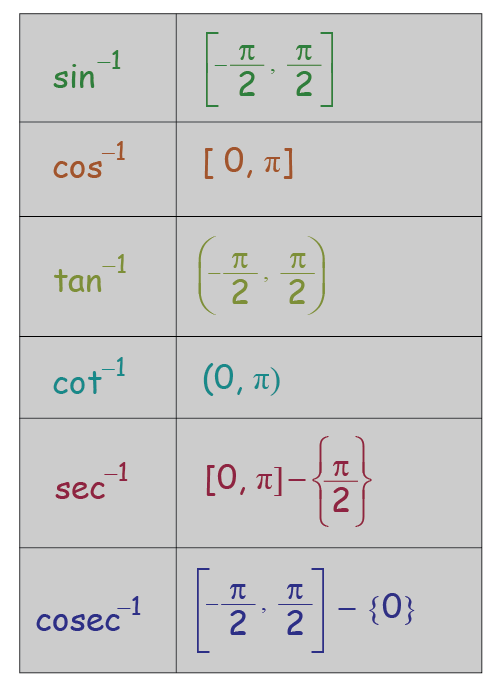## Cofunction Identities## Sum and Difference Identities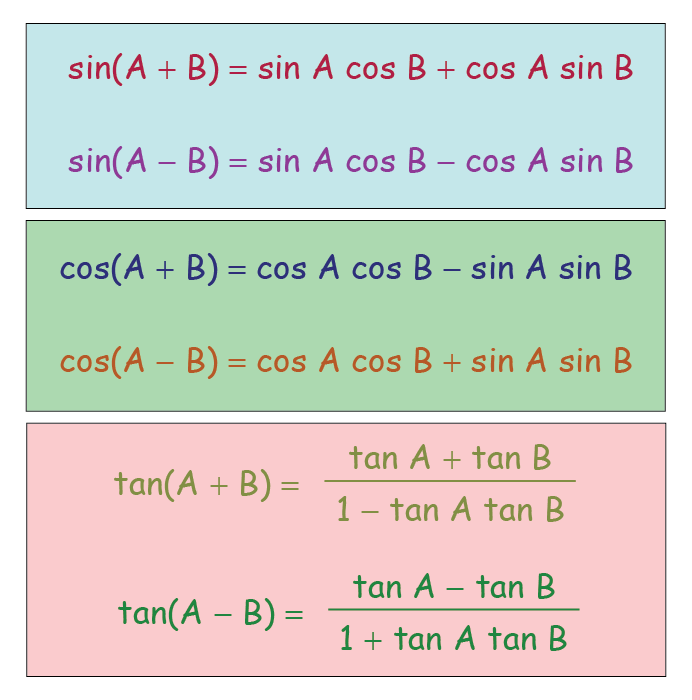## Double Angle Identities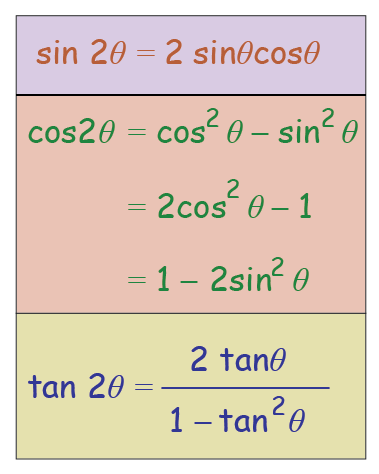## Half-angle identities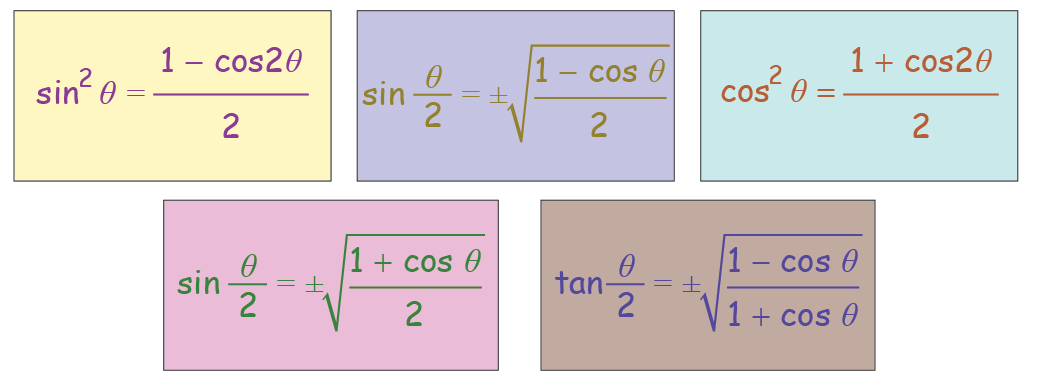## Heron’s Formula

The area of a triangle in terms of its semi-perimeter and side lengths can be written as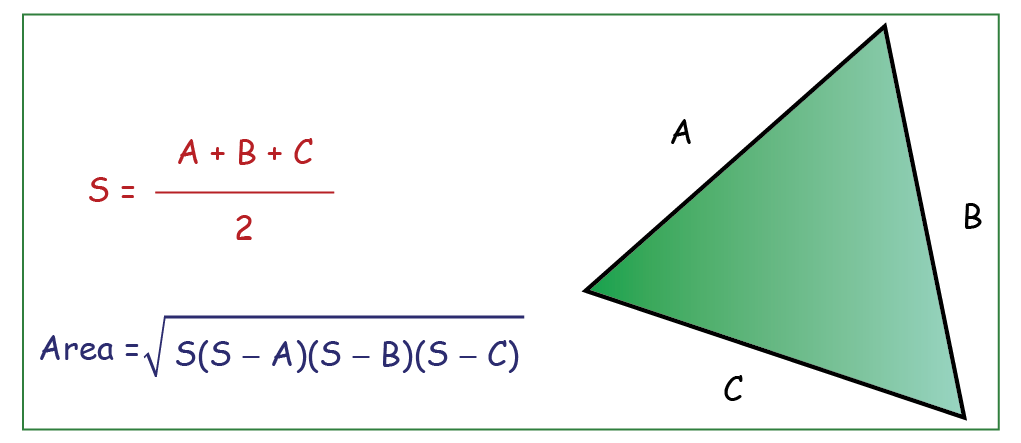## Sine Law

The sine law says that the sides of a triangle are proportional to the sines of the angles opposite to them.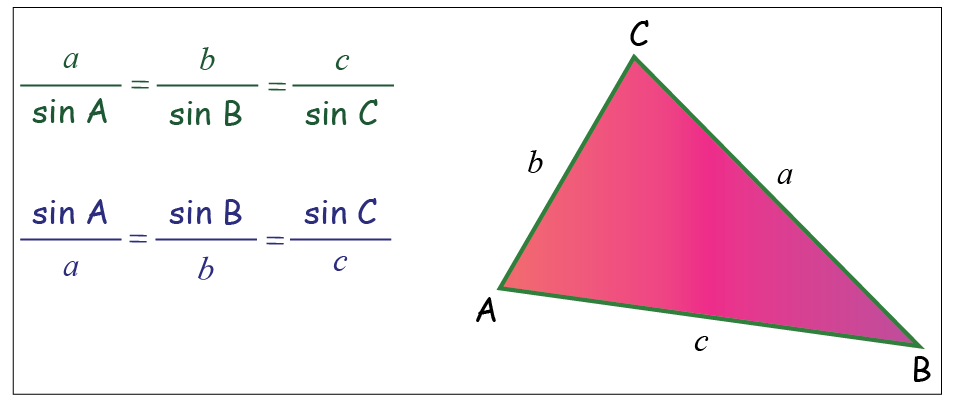## Cosine Law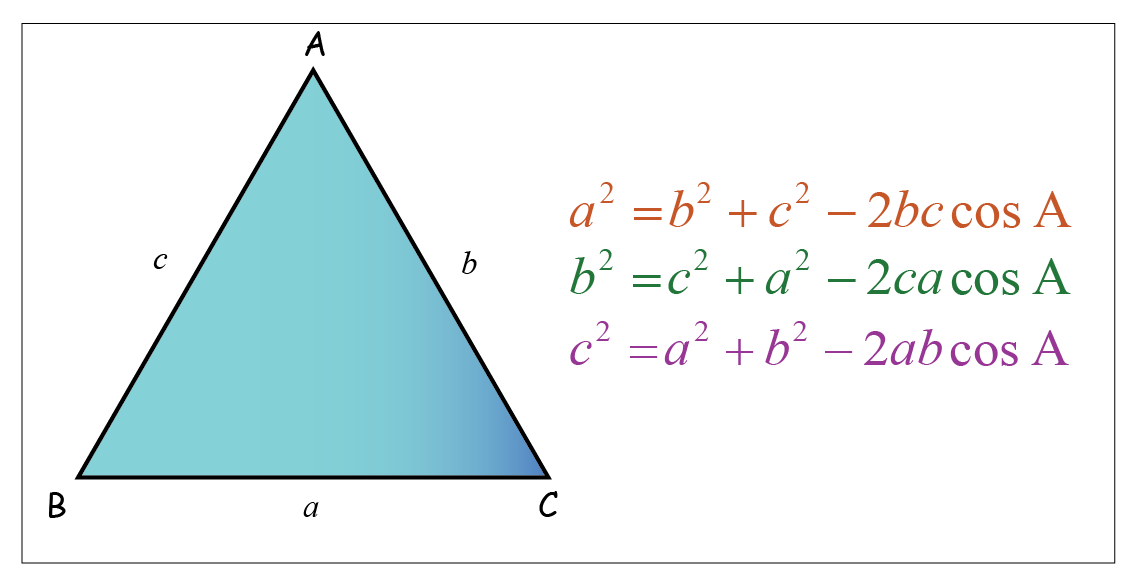More Important Topics
Numbers
Algebra
Geometry
Measurement
Money
Data
Trigonometry
Calculus
More Important Topics
Numbers
Algebra
Geometry
Measurement
Money
Data
Trigonometry
Calculus
Learn from the best math teachers and top your exams

• Live one on one classroom and doubt clearing
• Practice worksheets in and after class for conceptual clarity
• Personalized curriculum to keep up with school